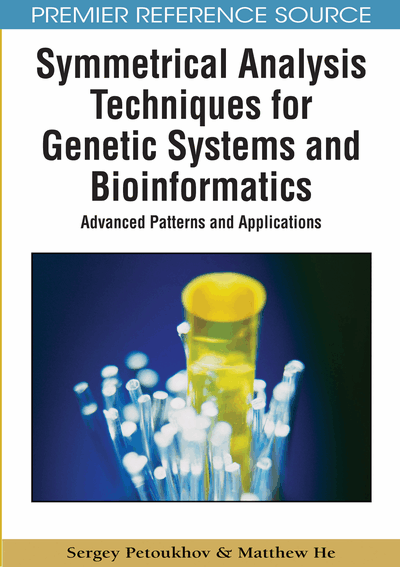# Genetic System, Fibonacci Numbers, and Phyllotaxis Laws

Sergey Petoukhov (Russian Academy of Sciences, Russia) and Matthew He (Nova Southeastern University, USA)
DOI: 10.4018/978-1-60566-124-7.ch010
Available
\$37.50
No Current Special Offers

## Abstract

This chapter describes data suggesting a connection between matrix genetics and one of the most famous branches of mathematical biology: phyllotaxis laws of morphogenesis. Thousands of scientific works are devoted to this morphogenetic phenomenon, which relates with Fibonacci numbers, the golden section, and beautiful symmetrical patterns. These typical patterns are realized by nature in a huge number of biological bodies on various branches and levels of biological evolution. Some matrix methods are known for a long time to simulate in mathematical forms these phyllotaxis phenomena. This chapter describes connections of the famous Fibonacci (2x2)-matrices with genetic matrices. Some generalizations of the Fibonacci matrices for cases of (2nx2n)-matrices are proposed. Special geometrical invariants, which are connected with the golden section and Fibonacci numbers and which characterize some proportions of human and animal bodies, are described. All these data are related to matrices of the genetic code in some aspects.
Chapter Preview
Top

## Introduction Background

The complexity of biological objects complicates the creation of appropriate mathematical simulators of their morphogenetic and other features. But one phenomenon has been famous in the field of biological morphology for a long time, which allows one to create non-trivial mathematical simulators. It is a morphogenetic phenomenon of phyllotaxis or a phenomenon of regular dispositions of leaves and some other parts in configurations of biological bodies. This inherited phenomenon is connected with Fibonacci numbers and with the golden section and it is observed at very various levels and branches of biological evolution (Adler, 1974, 1990; Bowman, Eshed, & Baum, 2002; Clark, 2001; Douady, & Couder, 1992; Jean, 1995; Lee, & Levitov, 1998; Stieger, Reinhardt, & Kuhlemeier, 2002; Thompson, 1942; Waites, & Hudson, 1995). The task of understanding the questions of how and why genetic systems provide such regular dispositions of biological parts is one of the important ones in the field of mathematical and theoretical biology.

Matrix genetics reveals some new ways to study such questions because it involves a matrix approach and a matrix presentation of the golden section and of Fibonacci numbers. Simultaneously new forms of manifestations of phyllotaxis or quasi-phyllotaxis laws can be revealed on the bases of data of matrix genetics, because it deals, for example, with Hadamard matrices and with genetic multi-dimensional algebras, which can lead to new facts of manifestations of Fibonacci numbers and the golden section in biological morphogenesis. Some mathematical aspects arise in this field, which need their accurate investigations from different sides. Initial results of such investigations are presented in this chapter.

The main objectives of this chapter are investigations of possibilities of generalizations of Fibonacci matrices and of golden genomatrices, which are connected with inherited phyllotaxis laws and with many manifestations of the golden section in physiological phenomena. Mathematical properties of these generalizations should be investigated as well. The hypothesis is put forward that invariants of projective geometry, which are observed as ontogenetic invariants of the kinematic scheme of the inherited human body, are connected with peculiarities of the genetic code and with its Hadamard matrices.

A possible connection of the genetic matrices with invariants of projective geometry, which are observed as ontogenetic invariants of the kinematic scheme of the human body, is presented.

Biological phenomena of phyllotaxis are one of the most famous and popular phenomena in the field of mathematical biology. Thousands of scientific works are devoted to this morphogenetic phenomenon, which relates with Fibonacci numbers and the golden section (see for example the review in the book (Jean, 1995) with references including 1000 thematic sources approximately). It is important that these inherited phenomena are illustrated by a huge number of regular helical patterns of biological morphogenesis and they are described by means of simple mathematical laws. These phenomena and their patterns interest not only biologists, but also mathematicians and physicists (Douady, & Couder, 1992; Koch, & Meinhardt, 1994; Levitov, 1991a,b; Mandelbrot, 1983).

According to these phyllotaxis laws, pairs of Fibonacci numbers are realized at once at helical arrangement of leaves (or seeds) in plant bodies (shoots). These pairs belong to the sequences of the two following types:

{Qn= Fn+1/ Fn}: 2/1, 3/2, 5/3, 8/5, 13/8, …⇨ φ = (1+50.5)/2 = 1.618…,{Qn’’= Fn+2/ Fn}: 2/1, 3/1, 5/2, 8/3, 13/5,…⇨ φ2 = 2.618… (1)

## Complete Chapter List

Search this Book:
Reset